# How to Find the Smallest Value and Smallest Positive Value in Excel

Sometimes we want to find out the smallest positive value among a set of values. We should ignore the negative value and zero value when getting the smallest positive value. So we design different cases in this article to demonstrate how can we get the smallest positive value by different formulas.

## Returns the Smallest Value by Small Function

If there are all positive values for a set of values, we can use SMALL function to get the smallest value directly. See the table below.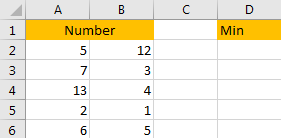There are a set of values, obliviously the smallest value is 1. But if we want use a formula to find out the smallest value, we can use SMALL function.

Step 1: In D2 cell, enter the formula =SMALL(A2:B6,1).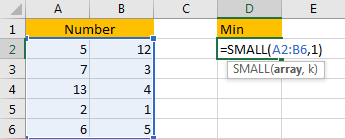Step 2: Click Enter to get the result.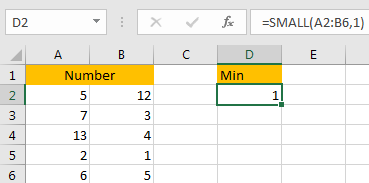## Returns the k-th Smallest Value by Small Function

In above case, we enter the formula =SMALL(A2:B6,1) then we can get the smallest value 1. For SMALL function, the parameter is SMALL(array,k), k is ‘Returns the k-th minimum value in the dataset’. So, we can change k number to get the k-th smallest value.

Follow above steps, in D2 cell change the formula to =SMALL(A2:B6,2). Then we can get the second smallest value 2.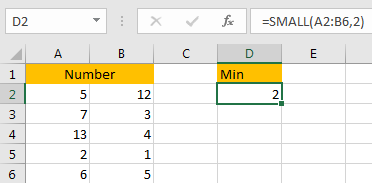## Returns the Smallest Positive Value by Small Function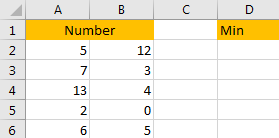Update above table a little. Replace a positive number with 0. So If we still use above SMALL function without any criteria, it will return 0 as the smallest value. So if we still want to use the SMALL function here, we need to count the number of zero value, then we need to add 1 base on the number of zero value.

Step 1: In D2 cell, enter the formula =SMALL(A2:B6,COUNTIF(A2:B6,0)+1), where COUNTIF function is used for counting the number of zero value.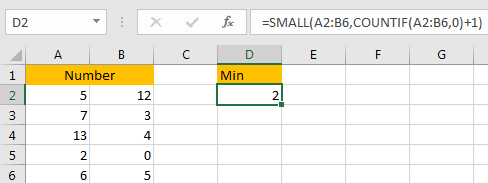Step 2: Let’s replace some values with zero values and check the formula again.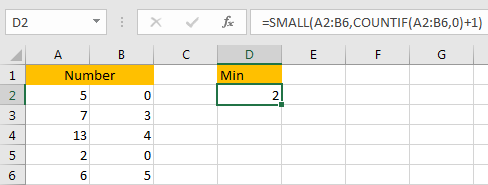## Returns the Smallest Positive Value by MIN Function

If we don’t want to add any criteria in SMALL formula to identify if zero value or negative value exists among the set of values, we can directly use MIN function.

See the table below, it contains both negative value and zero value.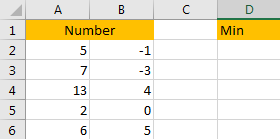Step 1: In D2 cell, enter the formula =MIN(IF(A2:B6>0,A2:B6)). IF function returns an array which satisfy the criteria ‘every value in the array >0’.

Step 2: Press control+shift+enter to returns value.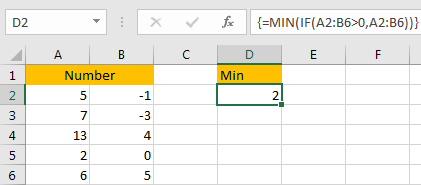### Related Functions

• Excel SMALL function
The Excel SMALL function returns the smallest numeric value from the numbers that you provided. Or returns the smallest value in the array.The syntax of the SMALL function is as below:=SMALL(array,nth) …
• Excel IF function
The Excel IF function perform a logical test to return one value if the condition is TRUE and return another value if the condition is FALSE. The IF function is a build-in function in Microsoft Excel and it is categorized as a Logical Function.The syntax of the IF function is as below:= IF (condition, [true_value], [false_value])….
• Excel COUNTIF function
The Excel COUNTIF function will count the number of cells in a range that meet a given criteria. This function can be used to count the different kinds of cells with number, date, text values, blank, non-blanks, or containing specific characters.etc.= COUNTIF (range, criteria)…
• Excel MIN function
The Excel MIN function returns the smallest numeric value from the numbers that you provided. Or returns the smallest value in the array.The MIN function is a build-in function in Microsoft Excel and it is categorized as a Statistical Function.The syntax of the MIN function is as below:= MIN(num1,[num2,…numn])….
Related Posts

How to Sum the Smallest N Values in Excel

Sometimes we may want to sum the first smallest N numbers in a range in Excel. In this article, we will show you the method of “SUM the Smallest N Numbers” by a simple formula which consist of SUMPRODUCT and ...

How to Create Dynamical Drop-Down List and Sort by Alphabetical Order in Excel

In our daily work we may need to create a dynamical dropdown list and sort all values by alphabetical order. To create a dropdown list like this, we need to apply some built-in features like ‘Define Name’ and ‘Data Validation’ ...

How to Get the First, 2nd or Nth Match Using Vlookup/Index/Match

This post will guide you how get the nth matching values with VLOOKUP function in Excel. How do I find the nth match value with Index/Match formula in Excel. How to find the first, second, third or nth matching value ...

Ignoring Blank or Zero Cells with Conditional formatting

This post will guide you how to make conditional formatting ignore blank cells or zero cell in Excel. How do I force blank cells or zero cells to be ignored in conditional formatting in Excel. How to ignore blank cells ...

Find the Earliest and Latest Date in a Range of Dates in Excel

This post will guide you how to find the earliest date in a range of dates in Excel. How do I get the earliest and latest date in a range with a formula in Excel. How to find the highest ...

Find Closest Value or Nearest Value in a Range in Excel

This post will guide you how to find the closest value or nearest value in a range of cells or in a column in Excel. How do I find the closest match in a range to a given lookup value ...

Find Missing Numbers in a Sequence in Excel

This post will guide you how to find missing numbers in a sequence with a formula in Excel. How do I identify missing numbers in a consecutive series in Excel. How to find missing serial number in Excel 2013/2016. Find ...

Copy and Paste Only Non-blank Cells

This post will guide you how to copy and paste only non-blank cells in Excel 2013/2016. How do I copy only non blank cells with VBA code in Excel. Or how to get non-blank cells from a range of cells ...

VLOOKUP Return Multiple Values Horizontally

This post will guide you how to vlookup a value and then return multiple corresponding values horizontally in Excel. How do I lookup a value and return multiple matched values in the same row in Excel. Or how to return ...

How to Find the nth Smallest Value

This post explains that how to get the nth smallest value in a single column, or single row or an array list in excel. How to extract relative value in the same row based on the position of the nth ...

Sidebar